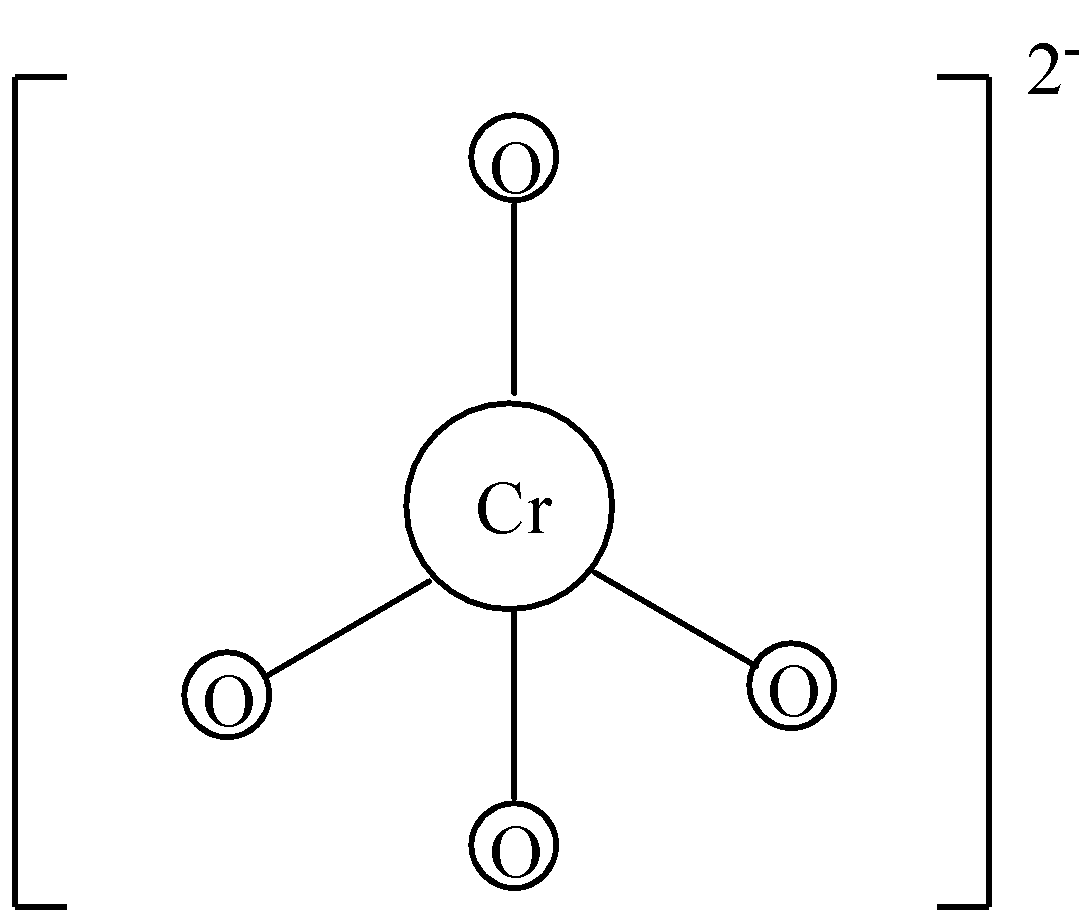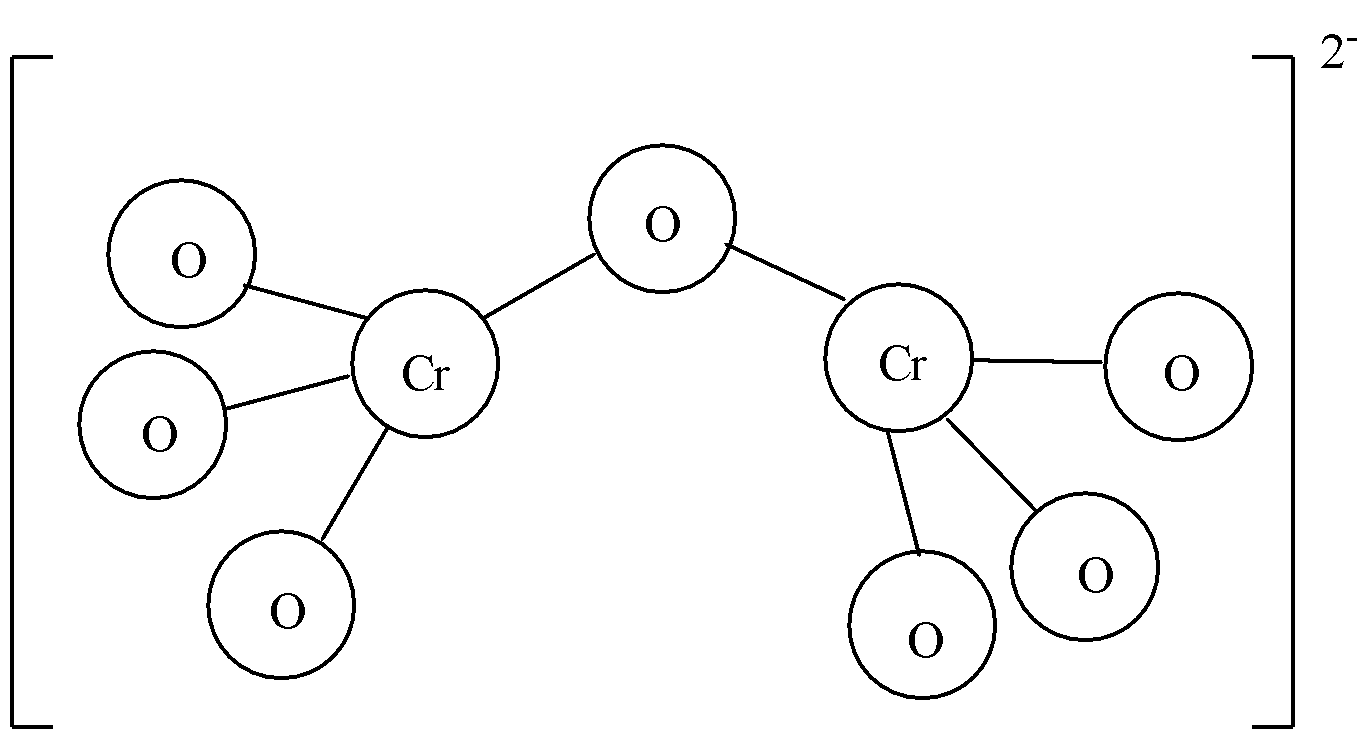Courses
Courses for Kids
Free study material
Free LIVE classes
MoreLIVE
Join Vedantu’s FREE Mastercalss

# The ionic charges on chromate ion and dichromate ion respectively are:(a)-2, -2(b)-3, -2(c)-2, -4(d)-4, -2Verified
335.1k+ views
Hint: Ionic charge is the charge on an ion. Ionic charge is due to the gain or loss of one or more electrons from an atom or a group of atoms.

As mentioned above, ionic charge is the charge on an ion. Let us discuss the two ions mentioned in the question.
-Chromate ion or chromate anion is present in chromate salts. When chromate salt dissociates, we get chromate ions. It consists of one chromium and four oxygen atoms. It is written as $CrO_{4}^{2-}$. The charge on the chromate ion is -2. The oxidation state of chromium in chromate ion is +6 and the oxidation state of oxygen is -2. There are four oxygen atoms, so we have to multiply -2 with four to get -8. By adding the oxidation state of chromium and total oxidation state of oxygen, we get -2. So, the ionic charge on chromate ions is -2.-Dichromate ions are obtained from dissociation of dichromate salts. As the name suggests, there are two chromium atoms in dichromate ion. Dichromate is also an anion. It has an ionic charge of -2. We know that the oxidation state of chromium is +6 in dichromate. There are two chromium atoms, so we need to multiply +6 with two, we get +12. Dichromate anion consists of seven oxygen atoms and oxidation state of oxygen is -2. Multiplying seven with -2 we get -14. Adding the total oxidation state of chromium and oxygen, we get -2. So, the ionic charge on dichromate ions is -2.Thus, both chromate ion and dichromate ion have ionic charge -2. The correct answer to the question is option (a).
Note: In chromate ion and dichromate ion, the oxidation state of chromium is +6. We already know the oxidation state of oxygen is -2, we can multiply the total number of oxygen present in the molecule with its oxidation state and add it with the oxidation state of chromate ion or dichromate ion. We obtain the total ionic charge of the molecule.
Last updated date: 26th Sep 2023
Total views: 335.1k
Views today: 7.35k